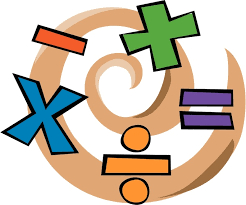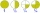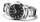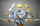# Write 3

Write a real world problem involving the multiplication of a fraction and a whole number with a product that is between 8 and 10 then solve the problem

x =  9

### Step-by-step explanation:Did you find an error or inaccuracy? Feel free to write us. Thank you!Tips to related online calculators
Need help to calculate sum, simplify or multiply fractions? Try our fraction calculator.

## Related math problems and questions:

• Product of two fractionsProduct of two fractions is 9 3/5 . If one of the fraction is 9 3/7. Find the other fraction.
• Two numbers and its productThe product of two numbers are 2/3. If on of them is 1/10, what is the other?
• Divide 11Divide the product of 4 and 5/8 by 1 1/2. Write your answer as a mixed number.
• Product increasedWhen the product of 2/3 and 6/10 is increased by 2/5, the result is?
• JonahJonah has an 8-pound bag of potting soil. He divides it evenly among 5 flowerpots. How much soil is in each pot? Show your answer as a fraction or mixed number. Solve this problem any way you choose.
• Evaluate 17Evaluate 2x+6y when x=- 4/5 and y=1/3. Write your answer as a fraction or mixed number in simplest form.
• Algebra problemThis is algebra. Let n represent an unknown number. 1. Eight more than the number n 2. Three times the number n 3. The product of the number n and eight 4. Three less than the number n 5. Three decreased by the number n
• FractionsSort fractions z1 = (6)/(11); z2 = (10)/(21); z3 = (19)/(22) by its size. Result write as three serial numbers 1,2,3.
• Fraction of a NumberIf 1/2 of 1/3 of 1/4 of 1/5 of a number is 2.5. what is the number?
• DozenWhat is the product of 26 and 5? Write the answer in an Arabic numeral. Add up the digits. How many of this is in a dozen? Divide #114 by this
• ArelliArelli had 20 minutes to do a three-problem quiz. She spent 9 7/10 minutes on question A and 3 2/5 minutes on question B. How much time did she have left for question C? Solve on paper to find the answer as a fraction.
• MinutesWrite as fraction in basic form which part of the week is 980 minutes.
• Divide 13Divide. Simplify your answer and write as an improper fraction or whole number. 14÷8/3Why does 1 3/4 + 2 9/10 equal 4.65? How do you solve this?Calculate the product of the sum and difference of numbers -7 and -2.Rewrite mixed numbers, so the fractions have the same denominator: 5 1/5 - 2 2/3The product of two functions is 10. If one of them is 2 1/3, find the other one.